# Ratio Ratio Ratios and Rates Ratios and Rates

• Slides: 15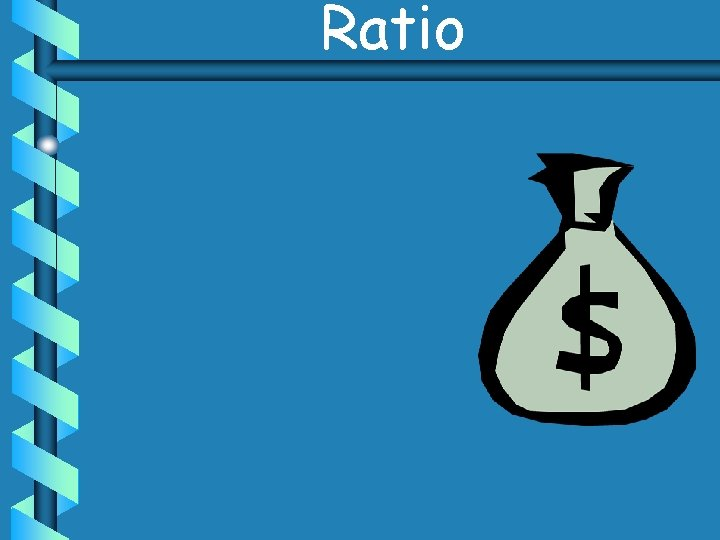RatioRatio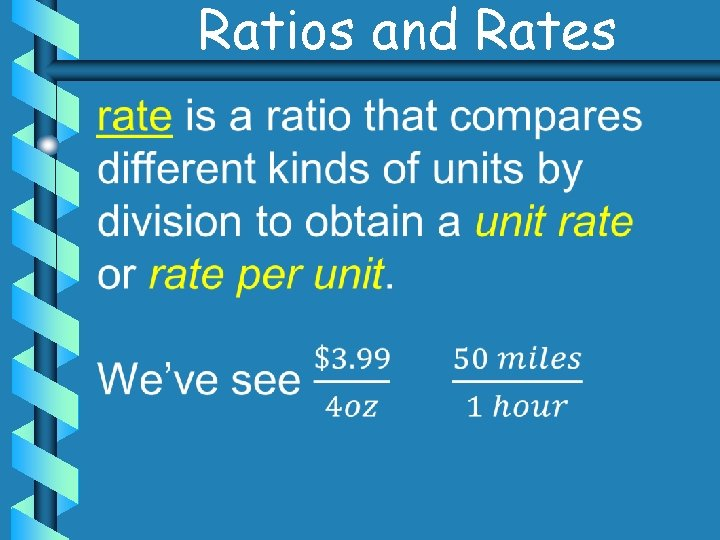Ratios and Rates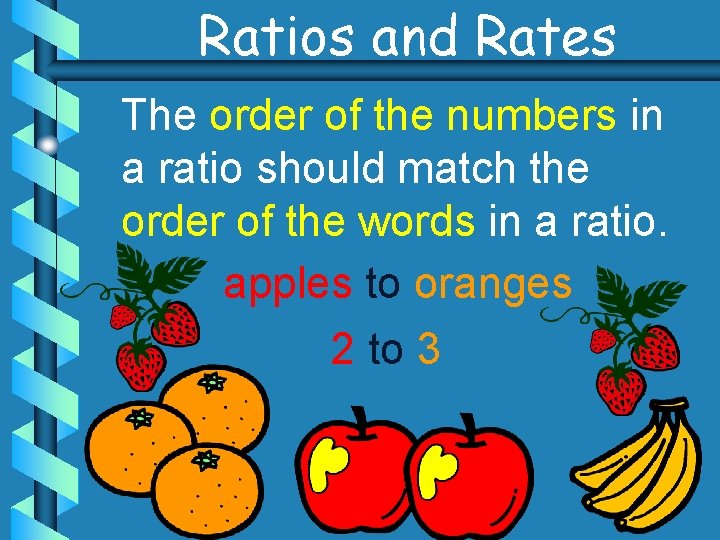Ratios and Rates The order of the numbers in a ratio should match the order of the words in a ratio. apples to oranges 2 to 3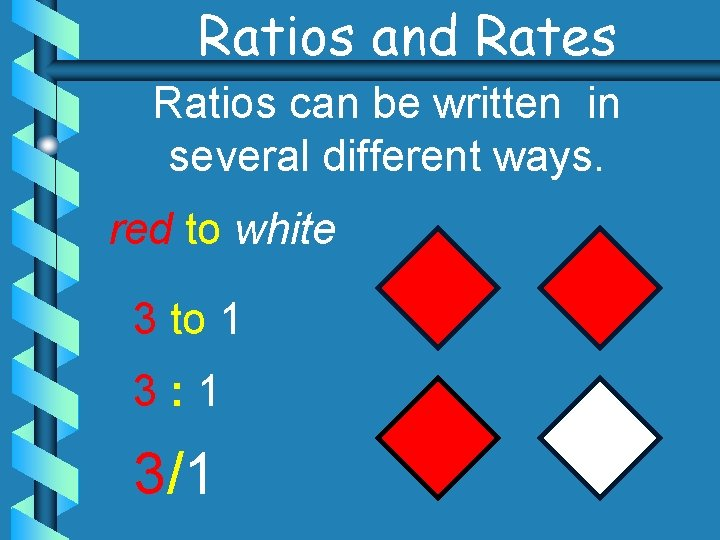Ratios and Rates Ratios can be written in several different ways. red to white 3 to 1 3: 1 3/1Ratios and Rates Ratios can show comparisons between: part to part red to green 1 to 2 green to red 2 to 1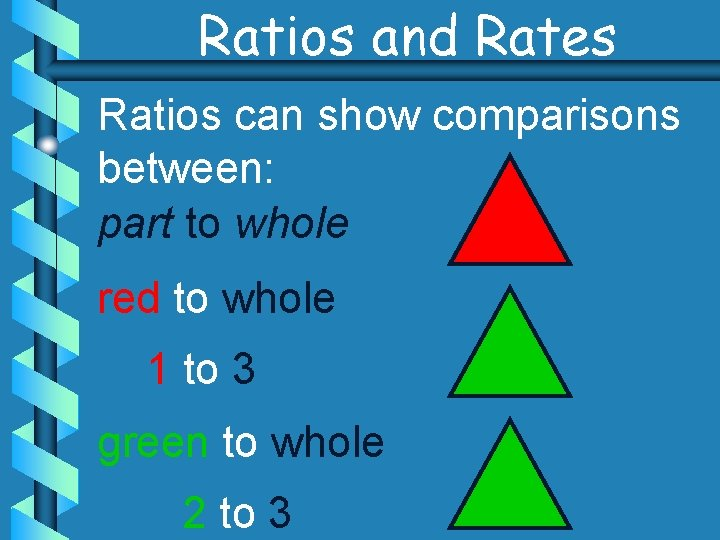Ratios and Rates Ratios can show comparisons between: part to whole red to whole 1 to 3 green to whole 2 to 3Ratios and Rates Ratios can show comparisons between: whole to part whole to red 3 to 1 whole to green 3 to 2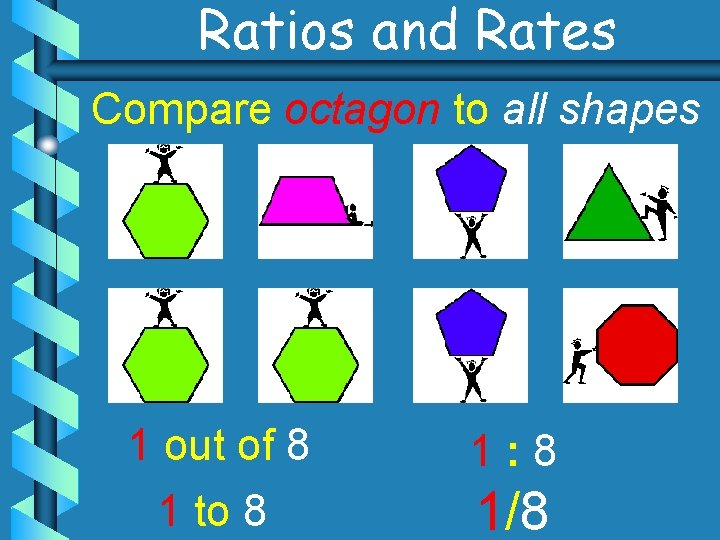Ratios and Rates Compare octagon to all shapes 1 out of 8 1 to 8 1: 8 1/8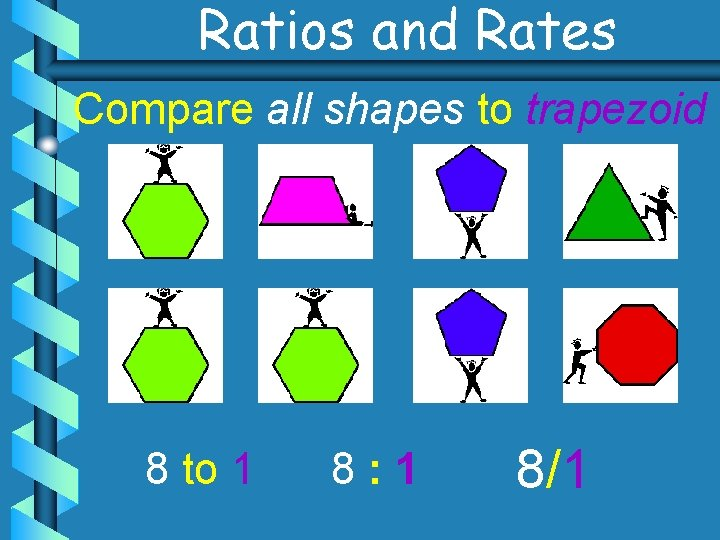Ratios and Rates Compare all shapes to trapezoid 8 to 1 8: 1 8/1Ratios and Rates Compare pentagons to hexagons 2 to 3 2: 3 2/3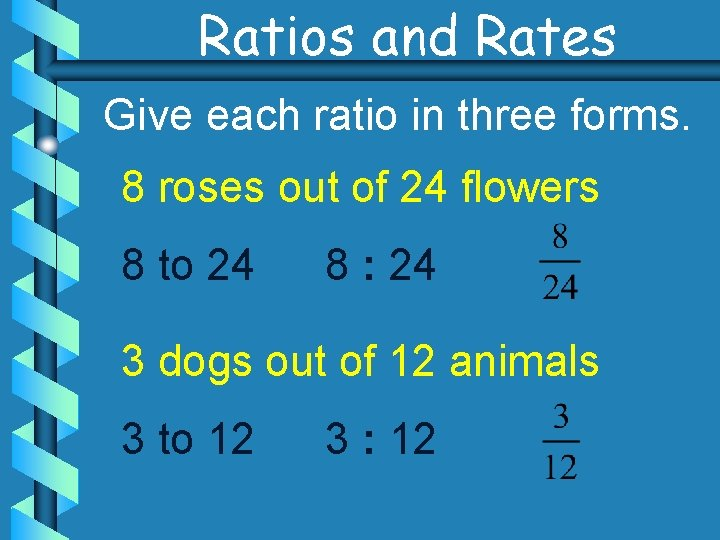Ratios and Rates Give each ratio in three forms. 8 roses out of 24 flowers 8 to 24 8 : 24 3 dogs out of 12 animals 3 to 12 3 : 12Ratios and Rates A ratio in fraction form can be expressed in simplest form by dividing the numerator and denominator by a common factor. ÷ 8 ÷ 3 = ÷ 8 = ÷ 3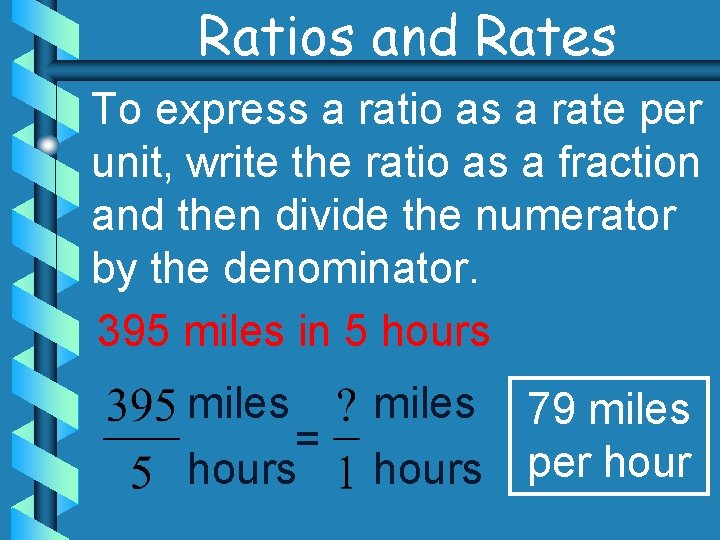Ratios and Rates To express a ratio as a rate per unit, write the ratio as a fraction and then divide the numerator by the denominator. 395 miles in 5 hours miles = hours miles 79 miles hours per hourRatios and Rates Express each ratio as a rate. 5 inches of rain in 30 days ÷ 5 = ÷ 5 inch of rain per day 120 words in 3 minutes = 40 words per minute